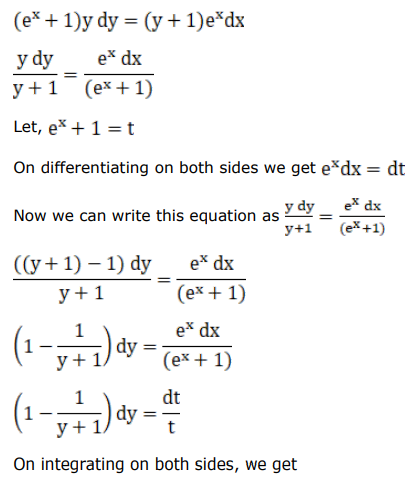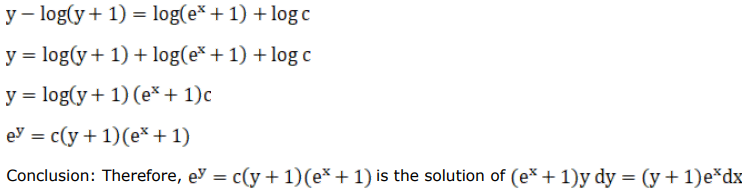# Mark against the correct answer in the following:Question:

Mark $(\sqrt{)}$ against the correct answer in the following:

The solution of the $\operatorname{DE}\left(e^{x}+1\right) y d y=(y+1) e^{x} d x$ is

A. $e^{y}=C\left(e^{x}+1\right)(y+1)$

B. $e^{y}=e^{x}+y+1$

C. $y=\left(e^{x}+1\right)(y+1)$

D. None of these

Solution: